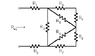# Finding equivalent resistance

Delta-One
I'm in a intro to circuits class for non-EE majors. One of the homework problems asks for the equivalent resistance.

See the attached file for a picture of this system.

I know the first step is to add resistors R4 and R5 (in series) as well as add resistors R6 and R7 (in series). But after that I'm stuck. I think I have to apply a delta (triangle) to Y transformation but I'm not sure how to proceed with it. Any help would be greatly welcomed.

P.S. Please don't tell me the rules for series and parallel circuits. I know what the are I just don't know which resistors to combine in this example.

#### Attachments

•pp1_5a.gif
2 KB · Views: 562

Homework Helper
I don't see how a delta y transformation would help you much. I would just start grouping resistors, R4 and R5, then R45 and R8, then the same thing on the bottom, etc.

Staff Emeritus
Resistors which are end-to-end can be combined as one in series, e.g. R45 = R4 + R5 and R67 = R6 + R7, as StatusX mentioned.

Resistors (or group) which share common nodes or junctions are combined in parallel.

Thus R45 is parallel with R8 giving Ra, and R67 is parallel with R9 giving Rb.

Then Ra and Rb are in series because they are end to end.

Rab is then in parallel with R2,

and the whole group (Rgroup) is in series with R1 and R3.

Req should have the form R1 + Rgroup + R3.